# RD Sharma Solutions for Class 11 Chapter 16 - Permutations Exercise 16.3

Each of the arrangements, which can be made by taking some or all of a number of things is termed as permutation. This exercise mainly deals with permutations and problems based on it. Examples are solved before the exercise wise problems to help increase knowledge about the fundamental concepts among students. Students can refer to the solutions pdf while solving the problems from RD Sharma textbook. Students can improve their problem solving and logical thinking abilities by using RD Sharma Class 11 Maths Solutions pdf, from the links given below.

## Download the pdf of RD Sharma Solutions for Class 11 Maths Exercise 16.3 Chapter 16 – Permutations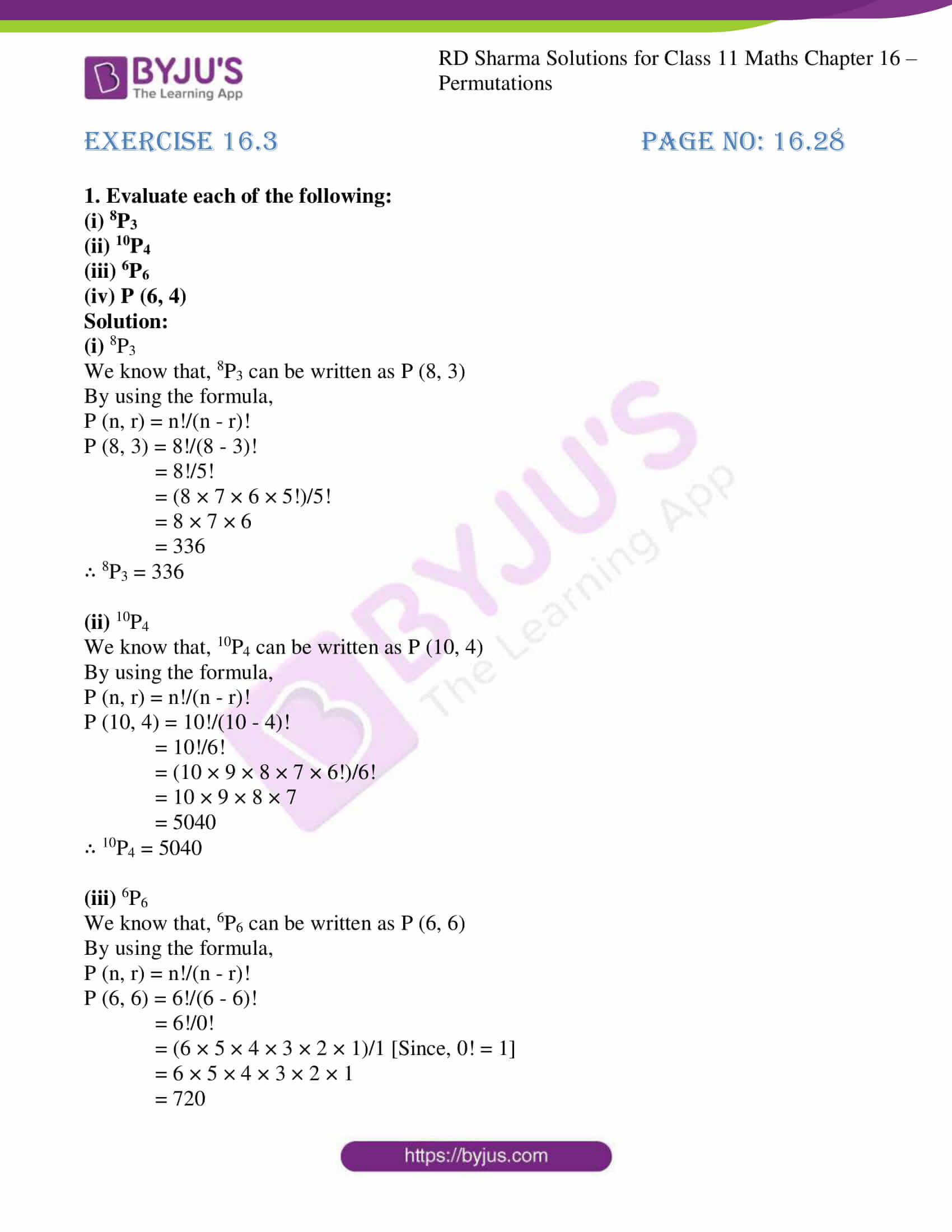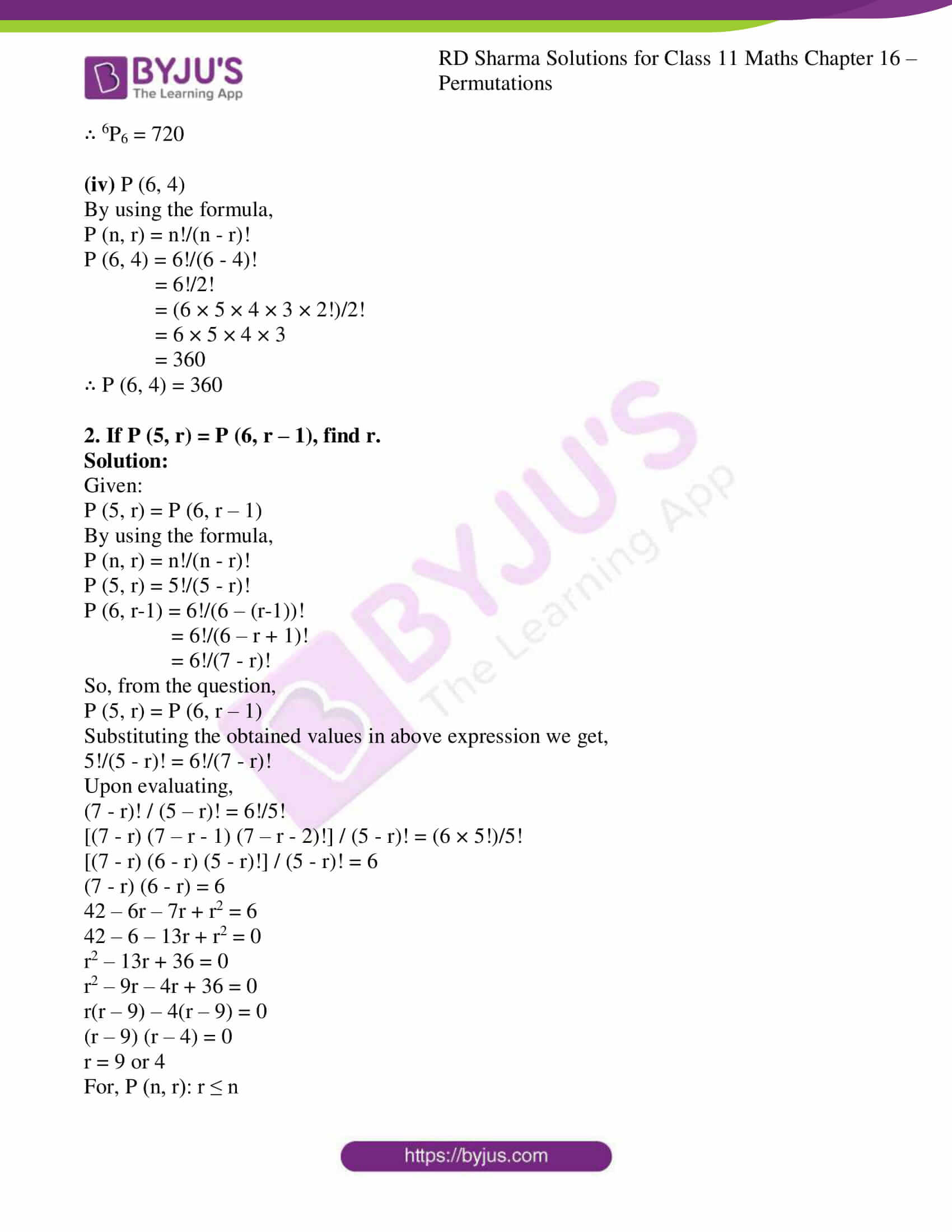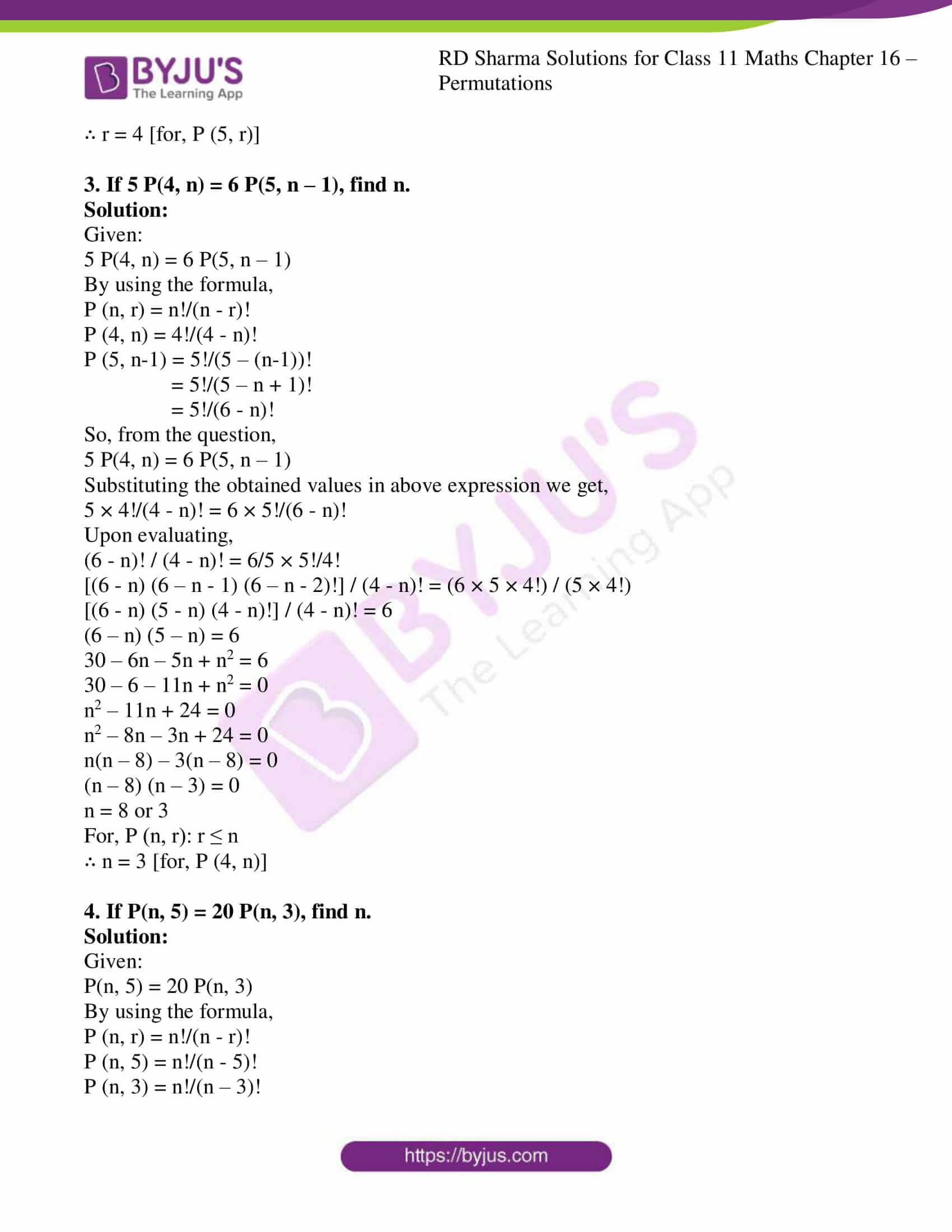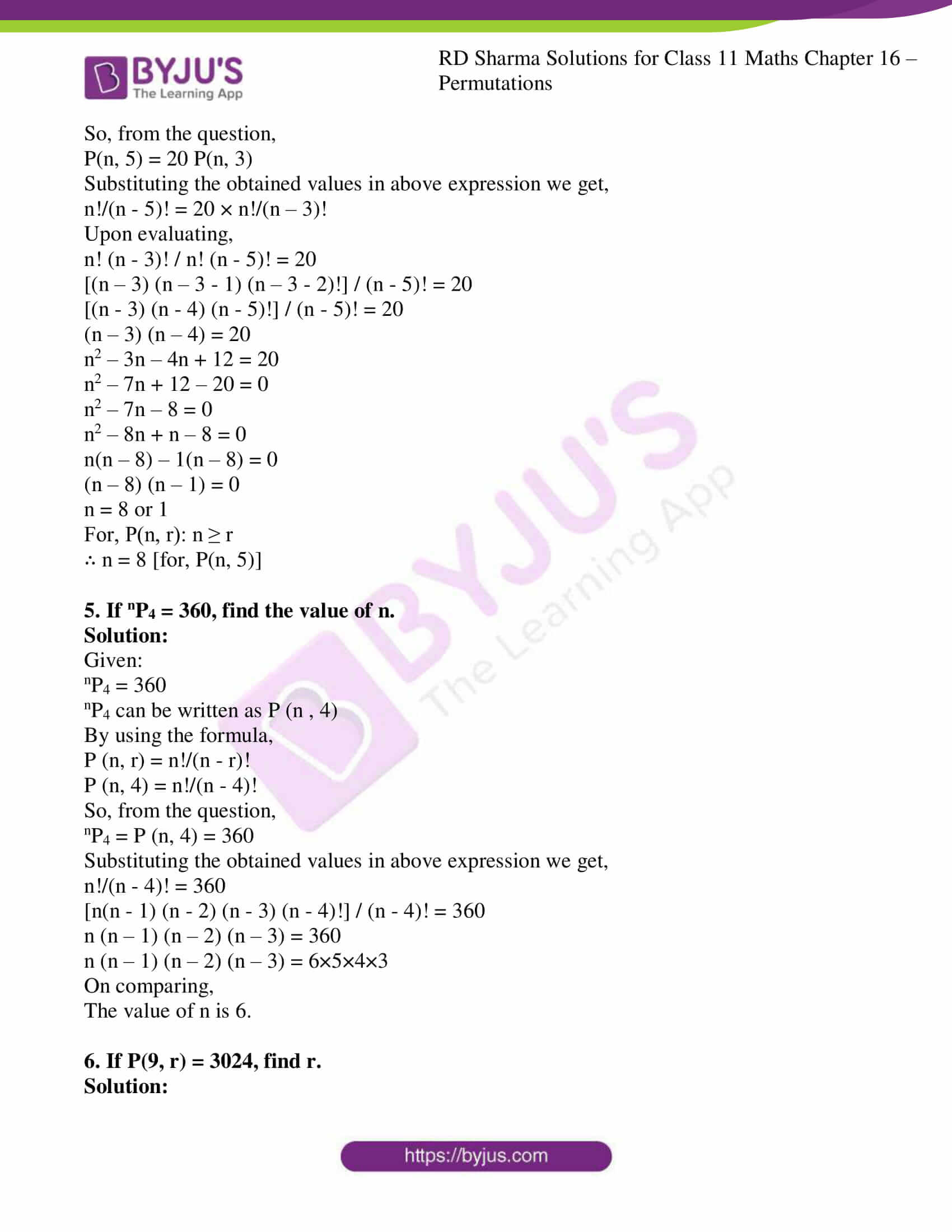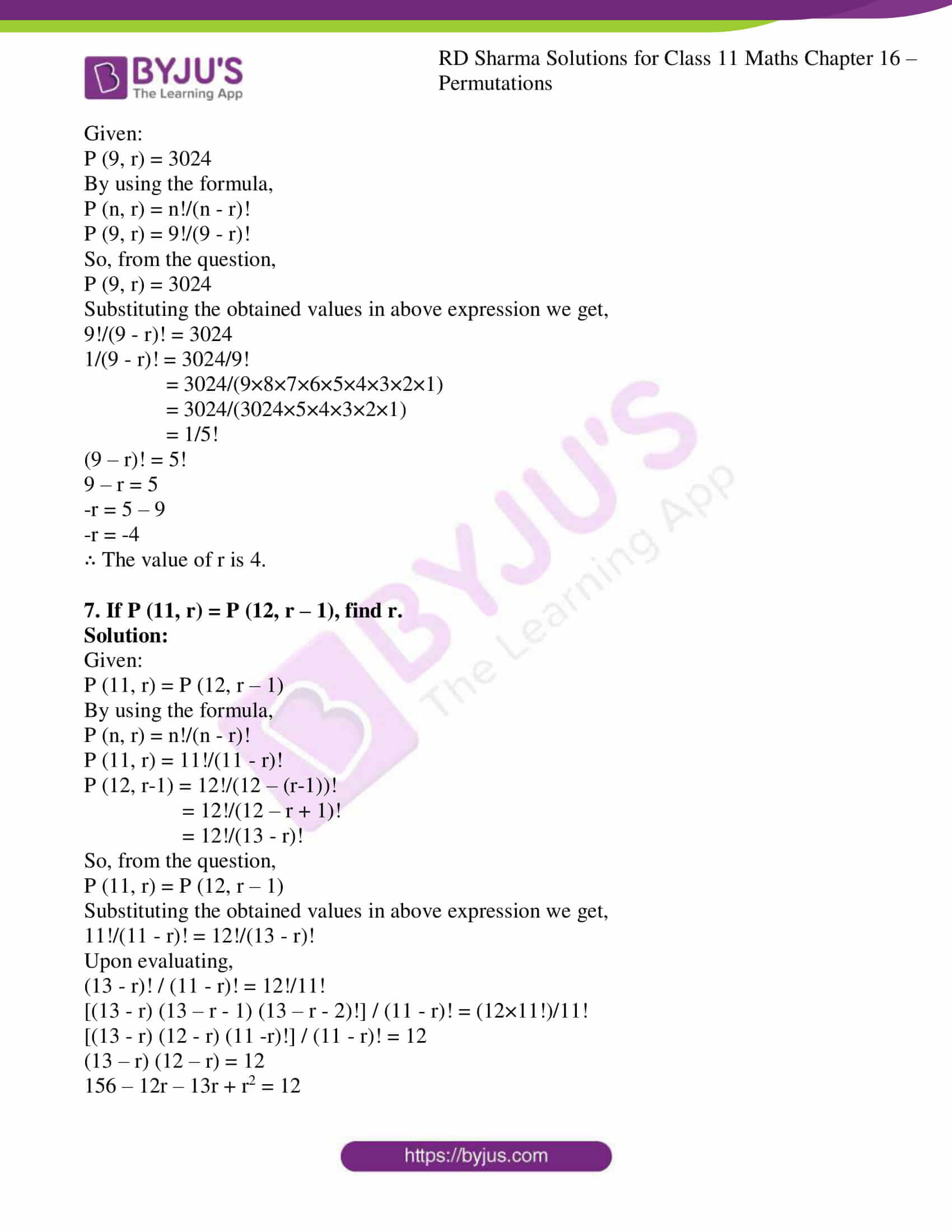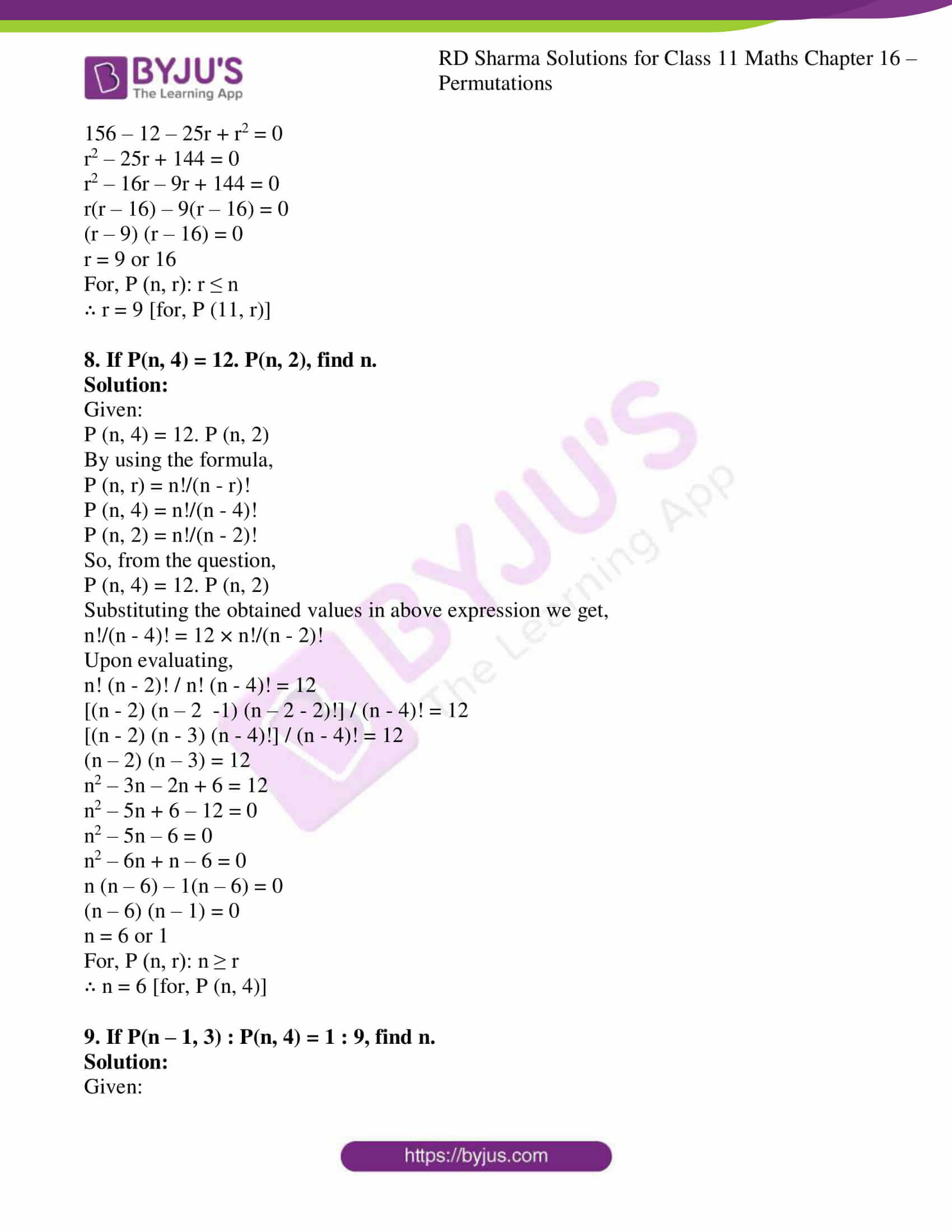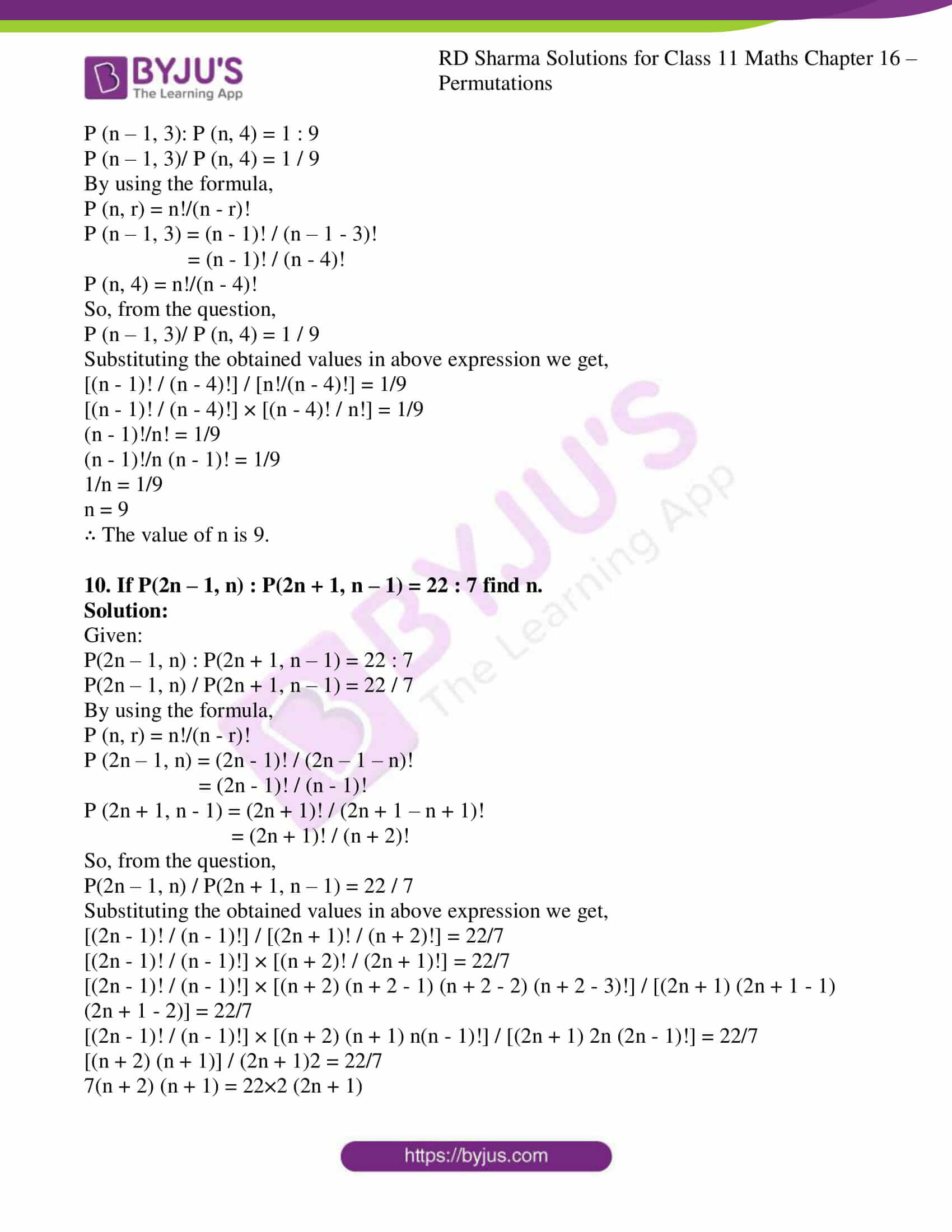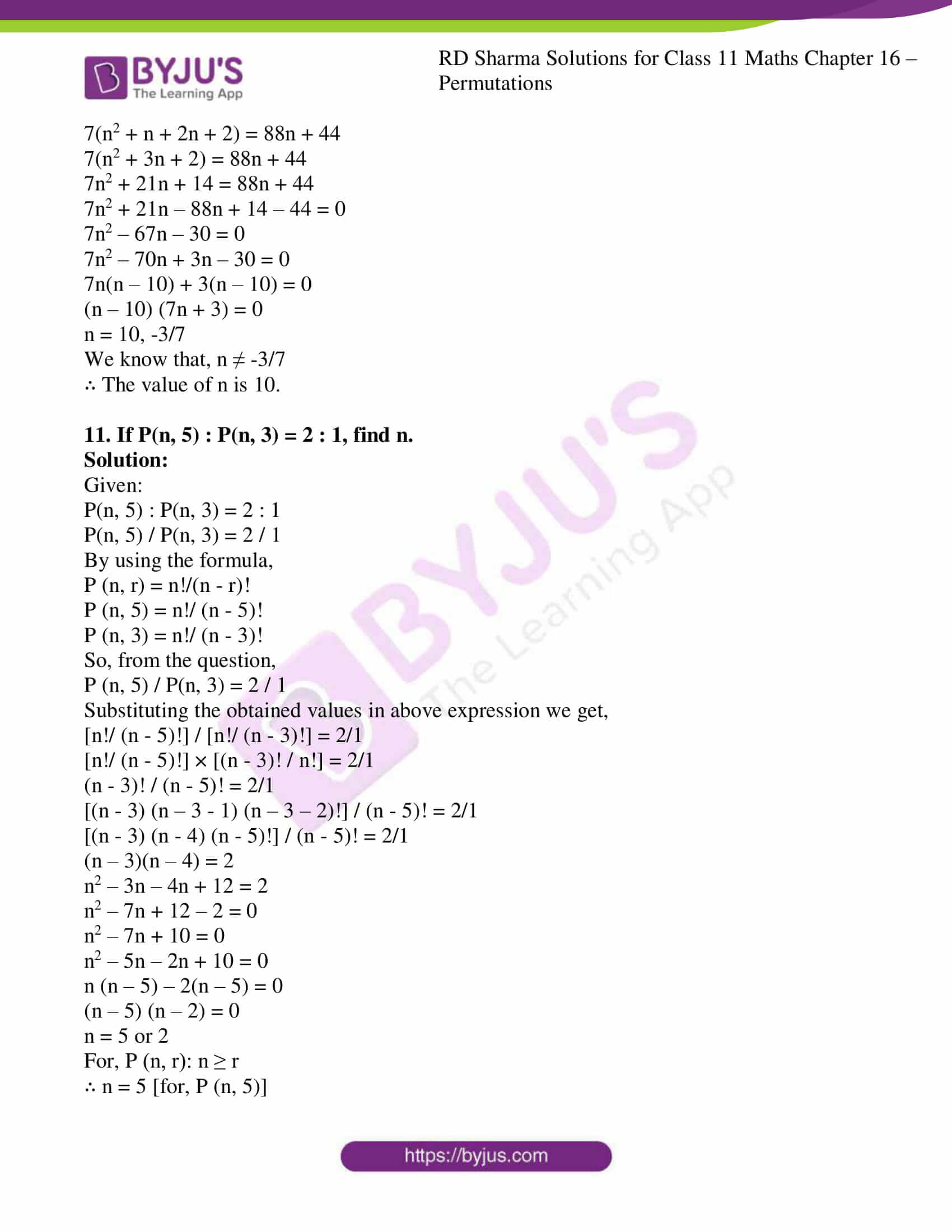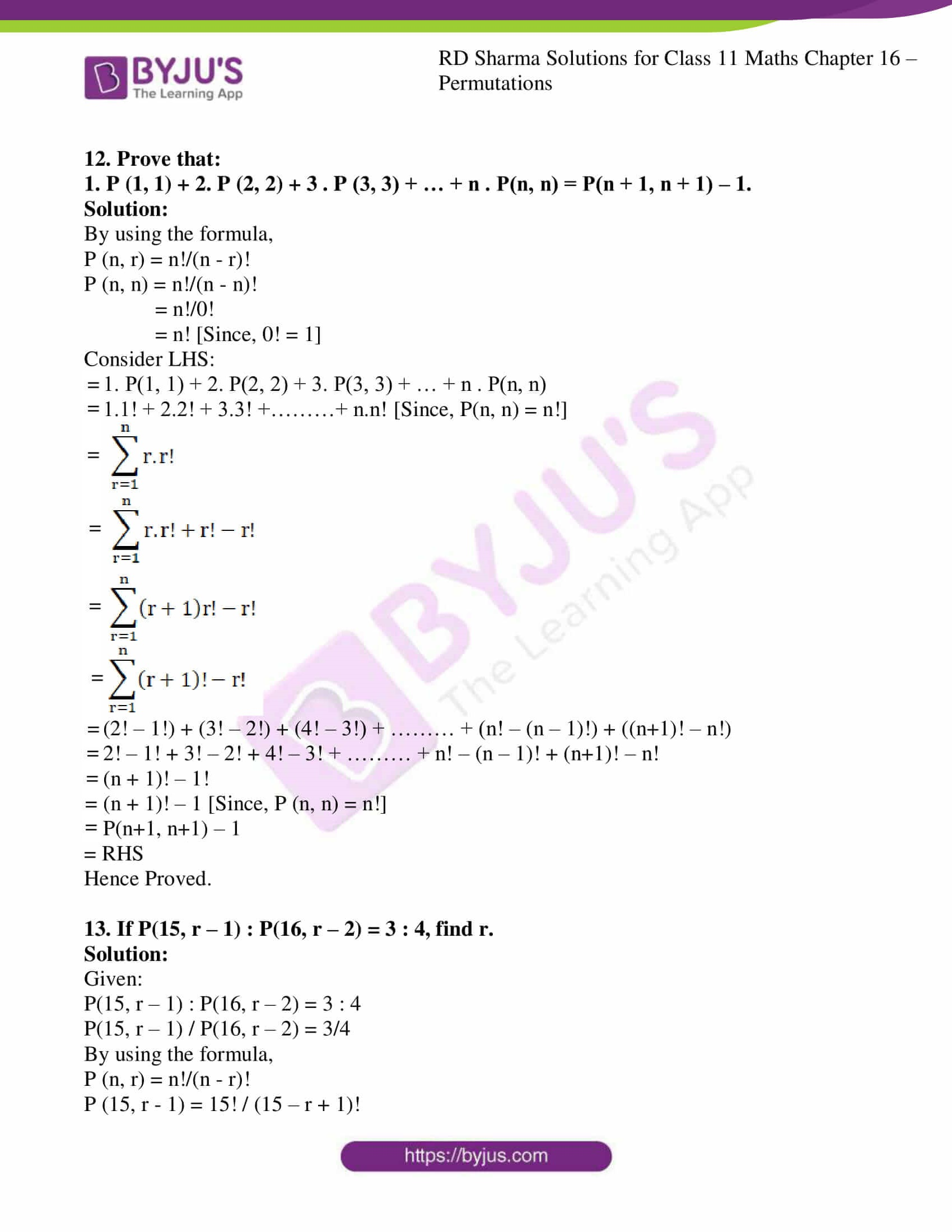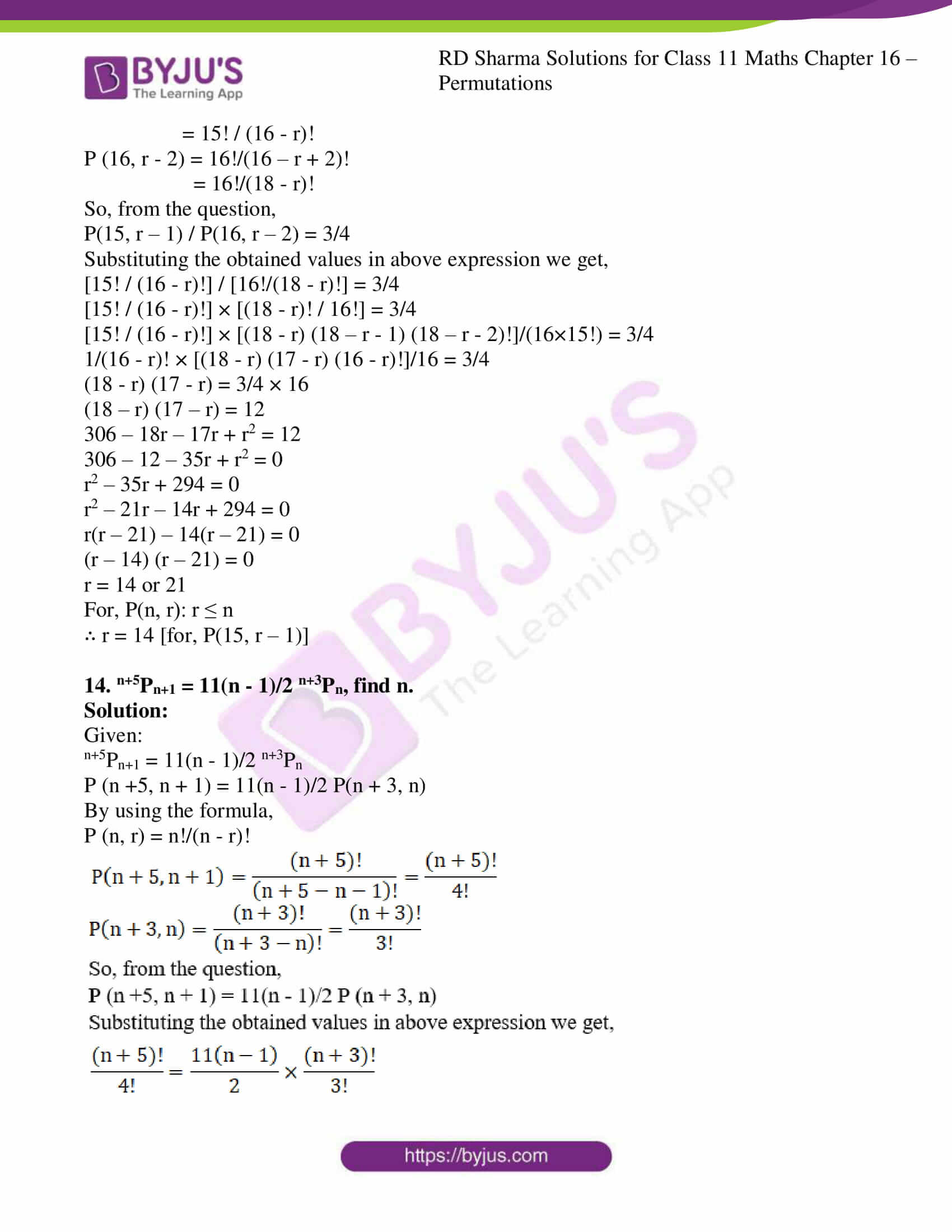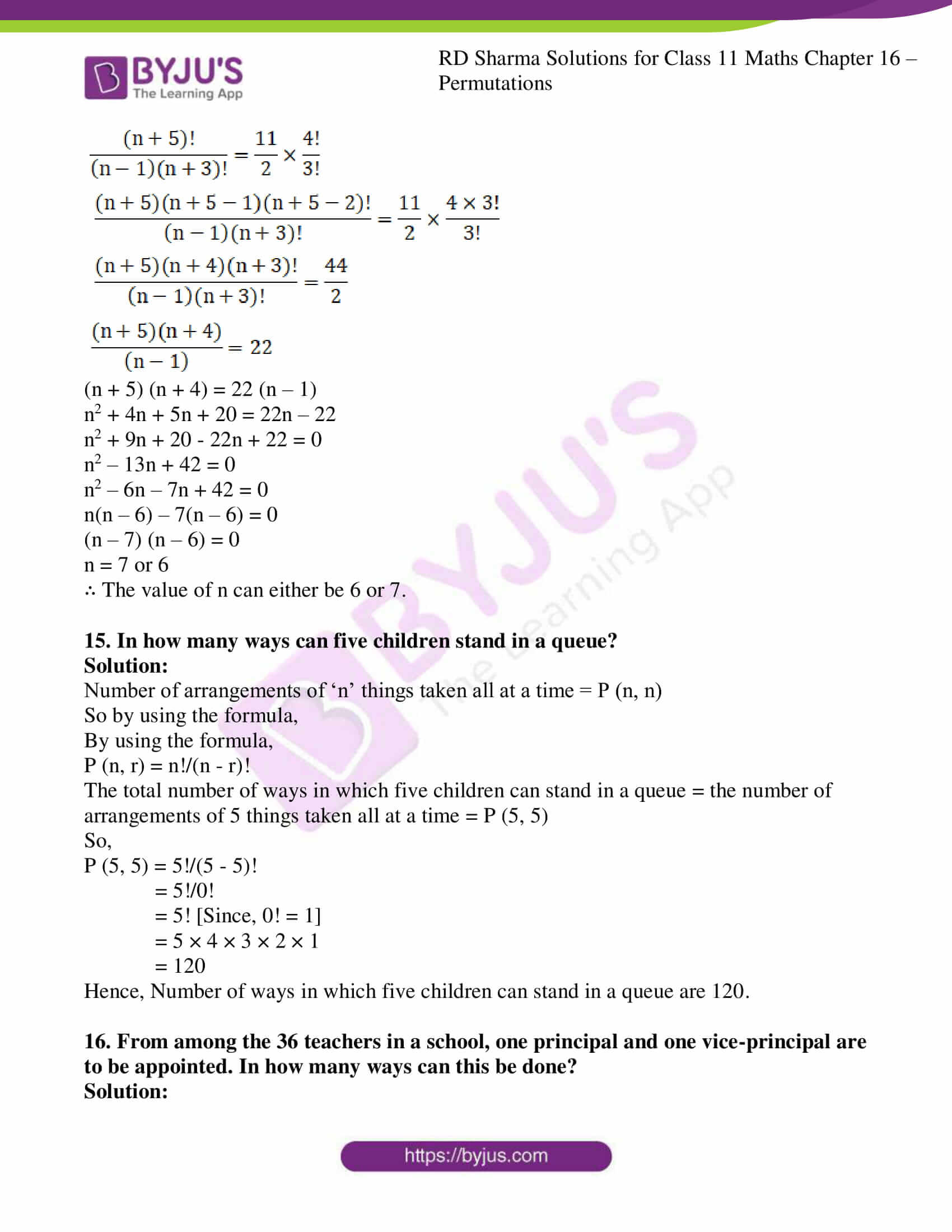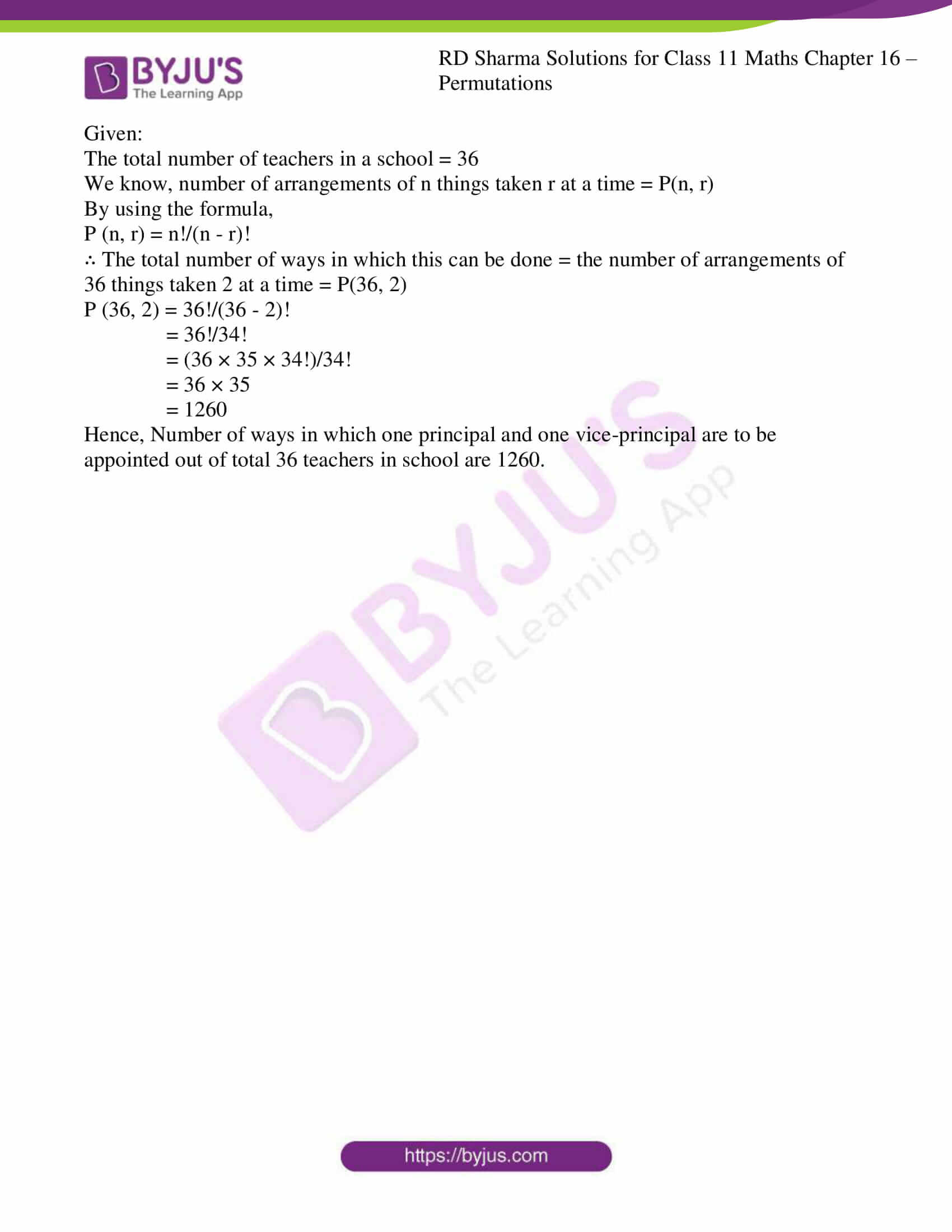### Also, access other exercises of RD Sharma Solutions for Class 11 Maths Chapter 16 – Permutations

Exercise 16.1 Solutions

Exercise 16.2 Solutions

Exercise 16.4 Solutions

Exercise 16.5 Solutions

### Access answers to RD Sharma Solutions for Class 11 Maths Exercise 16.3 Chapter 16 – Permutations

1. Evaluate each of the following:

(i) 8P3

(ii) 10P4

(iii) 6P6

(iv) P (6, 4)

Solution:

(i) 8P3

We know that, 8P3 can be written as P (8, 3)

By using the formula,

P (n, r) = n!/(n – r)!

P (8, 3) = 8!/(8 – 3)!

= 8!/5!

= (8 × 7 × 6 × 5!)/5!

= 8 × 7 × 6

= 336

8P3 = 336

(ii) 10P4

We know that, 10P4 can be written as P (10, 4)

By using the formula,

P (n, r) = n!/(n – r)!

P (10, 4) = 10!/(10 – 4)!

= 10!/6!

= (10 × 9 × 8 × 7 × 6!)/6!

= 10 × 9 × 8 × 7

= 5040

10P4 = 5040

(iii) 6P6

We know that, 6P6 can be written as P (6, 6)

By using the formula,

P (n, r) = n!/(n – r)!

P (6, 6) = 6!/(6 – 6)!

= 6!/0!

= (6 × 5 × 4 × 3 × 2 × 1)/1 [Since, 0! = 1]

= 6 × 5 × 4 × 3 × 2 × 1

= 720

6P6 = 720

(iv) P (6, 4)

By using the formula,

P (n, r) = n!/(n – r)!

P (6, 4) = 6!/(6 – 4)!

= 6!/2!

= (6 × 5 × 4 × 3 × 2!)/2!

= 6 × 5 × 4 × 3

= 360

∴ P (6, 4) = 360

2. If P (5, r) = P (6, r – 1), find r.

Solution:

Given:

P (5, r) = P (6, r – 1)

By using the formula,

P (n, r) = n!/(n – r)!

P (5, r) = 5!/(5 – r)!

P (6, r-1) = 6!/(6 – (r-1))!

= 6!/(6 – r + 1)!

= 6!/(7 – r)!

So, from the question,

P (5, r) = P (6, r – 1)

Substituting the obtained values in above expression we get,

5!/(5 – r)! = 6!/(7 – r)!

Upon evaluating,

(7 – r)! / (5 – r)! = 6!/5!

[(7 – r) (7 – r – 1) (7 – r – 2)!] / (5 – r)! = (6 × 5!)/5!

[(7 – r) (6 – r) (5 – r)!] / (5 – r)! = 6

(7 – r) (6 – r) = 6

42 – 6r – 7r + r2 = 6

42 – 6 – 13r + r2 = 0

r2 – 13r + 36 = 0

r2 – 9r – 4r + 36 = 0

r(r – 9) – 4(r – 9) = 0

(r – 9) (r – 4) = 0

r = 9 or 4

For, P (n, r): r ≤ n

∴ r = 4 [for, P (5, r)]

3. If 5 P(4, n) = 6 P(5, n – 1), find n.

Solution:

Given:

5 P(4, n) = 6 P(5, n – 1)

By using the formula,

P (n, r) = n!/(n – r)!

P (4, n) = 4!/(4 – n)!

P (5, n-1) = 5!/(5 – (n-1))!

= 5!/(5 – n + 1)!

= 5!/(6 – n)!

So, from the question,

5 P(4, n) = 6 P(5, n – 1)

Substituting the obtained values in above expression we get,

5 × 4!/(4 – n)! = 6 × 5!/(6 – n)!

Upon evaluating,

(6 – n)! / (4 – n)! = 6/5 × 5!/4!

[(6 – n) (6 – n – 1) (6 – n – 2)!] / (4 – n)! = (6 × 5 × 4!) / (5 × 4!)

[(6 – n) (5 – n) (4 – n)!] / (4 – n)! = 6

(6 – n) (5 – n) = 6

30 – 6n – 5n + n2 = 6

30 – 6 – 11n + n2 = 0

n2 – 11n + 24 = 0

n2 – 8n – 3n + 24 = 0

n(n – 8) – 3(n – 8) = 0

(n – 8) (n – 3) = 0

n = 8 or 3

For, P (n, r): r ≤ n

∴ n = 3 [for, P (4, n)]

4. If P(n, 5) = 20 P(n, 3), find n.

Solution:

Given:

P(n, 5) = 20 P(n, 3)

By using the formula,

P (n, r) = n!/(n – r)!

P (n, 5) = n!/(n – 5)!

P (n, 3) = n!/(n – 3)!

So, from the question,

P(n, 5) = 20 P(n, 3)

Substituting the obtained values in above expression we get,

n!/(n – 5)! = 20 × n!/(n – 3)!

Upon evaluating,

n! (n – 3)! / n! (n – 5)! = 20

[(n – 3) (n – 3 – 1) (n – 3 – 2)!] / (n – 5)! = 20

[(n – 3) (n – 4) (n – 5)!] / (n – 5)! = 20

(n – 3) (n – 4) = 20

n2 – 3n – 4n + 12 = 20

n2 – 7n + 12 – 20 = 0

n2 – 7n – 8 = 0

n2 – 8n + n – 8 = 0

n(n – 8) – 1(n – 8) = 0

(n – 8) (n – 1) = 0

n = 8 or 1

For, P(n, r): n ≥ r

∴ n = 8 [for, P(n, 5)]

5. If nP4 = 360, find the value of n.

Solution:

Given:

nP4 = 360

nP4 can be written as P (n , 4)

By using the formula,

P (n, r) = n!/(n – r)!

P (n, 4) = n!/(n – 4)!

So, from the question,

nP4 = P (n, 4) = 360

Substituting the obtained values in above expression we get,

n!/(n – 4)! = 360

[n(n – 1) (n – 2) (n – 3) (n – 4)!] / (n – 4)! = 360

n (n – 1) (n – 2) (n – 3) = 360

n (n – 1) (n – 2) (n – 3) = 6×5×4×3

On comparing,

The value of n is 6.

6. If P(9, r) = 3024, find r.

Solution:

Given:

P (9, r) = 3024

By using the formula,

P (n, r) = n!/(n – r)!

P (9, r) = 9!/(9 – r)!

So, from the question,

P (9, r) = 3024

Substituting the obtained values in above expression we get,

9!/(9 – r)! = 3024

1/(9 – r)! = 3024/9!

= 3024/(9×8×7×6×5×4×3×2×1)

= 3024/(3024×5×4×3×2×1)

= 1/5!

(9 – r)! = 5!

9 – r = 5

-r = 5 – 9

-r = -4

∴ The value of r is 4.

7. If P (11, r) = P (12, r – 1), find r.

Solution:

Given:

P (11, r) = P (12, r – 1)

By using the formula,

P (n, r) = n!/(n – r)!

P (11, r) = 11!/(11 – r)!

P (12, r-1) = 12!/(12 – (r-1))!

= 12!/(12 – r + 1)!

= 12!/(13 – r)!

So, from the question,

P (11, r) = P (12, r – 1)

Substituting the obtained values in above expression we get,

11!/(11 – r)! = 12!/(13 – r)!

Upon evaluating,

(13 – r)! / (11 – r)! = 12!/11!

[(13 – r) (13 – r – 1) (13 – r – 2)!] / (11 – r)! = (12×11!)/11!

[(13 – r) (12 – r) (11 -r)!] / (11 – r)! = 12

(13 – r) (12 – r) = 12

156 – 12r – 13r + r2 = 12

156 – 12 – 25r + r2 = 0

r2 – 25r + 144 = 0

r2 – 16r – 9r + 144 = 0

r(r – 16) – 9(r – 16) = 0

(r – 9) (r – 16) = 0

r = 9 or 16

For, P (n, r): r ≤ n

∴ r = 9 [for, P (11, r)]

8. If P(n, 4) = 12. P(n, 2), find n.

Solution:

Given:

P (n, 4) = 12. P (n, 2)

By using the formula,

P (n, r) = n!/(n – r)!

P (n, 4) = n!/(n – 4)!

P (n, 2) = n!/(n – 2)!

So, from the question,

P (n, 4) = 12. P (n, 2)

Substituting the obtained values in above expression we get,

n!/(n – 4)! = 12 × n!/(n – 2)!

Upon evaluating,

n! (n – 2)! / n! (n – 4)! = 12

[(n – 2) (n – 2 -1) (n – 2 – 2)!] / (n – 4)! = 12

[(n – 2) (n – 3) (n – 4)!] / (n – 4)! = 12

(n – 2) (n – 3) = 12

n2 – 3n – 2n + 6 = 12

n2 – 5n + 6 – 12 = 0

n2 – 5n – 6 = 0

n2 – 6n + n – 6 = 0

n (n – 6) – 1(n – 6) = 0

(n – 6) (n – 1) = 0

n = 6 or 1

For, P (n, r): n ≥ r

∴ n = 6 [for, P (n, 4)]

9. If P(n – 1, 3) : P(n, 4) = 1 : 9, find n.

Solution:

Given:

P (n – 1, 3): P (n, 4) = 1 : 9

P (n – 1, 3)/ P (n, 4) = 1 / 9

By using the formula,

P (n, r) = n!/(n – r)!

P (n – 1, 3) = (n – 1)! / (n – 1 – 3)!

= (n – 1)! / (n – 4)!

P (n, 4) = n!/(n – 4)!

So, from the question,

P (n – 1, 3)/ P (n, 4) = 1 / 9

Substituting the obtained values in above expression we get,

[(n – 1)! / (n – 4)!] / [n!/(n – 4)!] = 1/9

[(n – 1)! / (n – 4)!] × [(n – 4)! / n!] = 1/9

(n – 1)!/n! = 1/9

(n – 1)!/n (n – 1)! = 1/9

1/n = 1/9

n = 9

∴ The value of n is 9.

10. If P(2n – 1, n) : P(2n + 1, n – 1) = 22 : 7 find n.

Solution:

Given:

P(2n – 1, n) : P(2n + 1, n – 1) = 22 : 7

P(2n – 1, n) / P(2n + 1, n – 1) = 22 / 7

By using the formula,

P (n, r) = n!/(n – r)!

P (2n – 1, n) = (2n – 1)! / (2n – 1 – n)!

= (2n – 1)! / (n – 1)!

P (2n + 1, n – 1) = (2n + 1)! / (2n + 1 – n + 1)!

= (2n + 1)! / (n + 2)!

So, from the question,

P(2n – 1, n) / P(2n + 1, n – 1) = 22 / 7

Substituting the obtained values in above expression we get,

[(2n – 1)! / (n – 1)!] / [(2n + 1)! / (n + 2)!] = 22/7

[(2n – 1)! / (n – 1)!] × [(n + 2)! / (2n + 1)!] = 22/7

[(2n – 1)! / (n – 1)!] × [(n + 2) (n + 2 – 1) (n + 2 – 2) (n + 2 – 3)!] / [(2n + 1) (2n + 1 – 1) (2n + 1 – 2)] = 22/7

[(2n – 1)! / (n – 1)!] × [(n + 2) (n + 1) n(n – 1)!] / [(2n + 1) 2n (2n – 1)!] = 22/7

[(n + 2) (n + 1)] / (2n + 1)2 = 22/7

7(n + 2) (n + 1) = 22×2 (2n + 1)

7(n2 + n + 2n + 2) = 88n + 44

7(n2 + 3n + 2) = 88n + 44

7n2 + 21n + 14 = 88n + 44

7n2 + 21n – 88n + 14 – 44 = 0

7n2 – 67n – 30 = 0

7n2 – 70n + 3n – 30 = 0

7n(n – 10) + 3(n – 10) = 0

(n – 10) (7n + 3) = 0

n = 10, -3/7

We know that, n ≠ -3/7

∴ The value of n is 10.

11. If P(n, 5) : P(n, 3) = 2 : 1, find n.

Solution:

Given:

P(n, 5) : P(n, 3) = 2 : 1

P(n, 5) / P(n, 3) = 2 / 1

By using the formula,

P (n, r) = n!/(n – r)!

P (n, 5) = n!/ (n – 5)!

P (n, 3) = n!/ (n – 3)!

So, from the question,

P (n, 5) / P(n, 3) = 2 / 1

Substituting the obtained values in above expression we get,

[n!/ (n – 5)!] / [n!/ (n – 3)!] = 2/1

[n!/ (n – 5)!] × [(n – 3)! / n!] = 2/1

(n – 3)! / (n – 5)! = 2/1

[(n – 3) (n – 3 – 1) (n – 3 – 2)!] / (n – 5)! = 2/1

[(n – 3) (n – 4) (n – 5)!] / (n – 5)! = 2/1

(n – 3)(n – 4) = 2

n2 – 3n – 4n + 12 = 2

n2 – 7n + 12 – 2 = 0

n2 – 7n + 10 = 0

n2 – 5n – 2n + 10 = 0

n (n – 5) – 2(n – 5) = 0

(n – 5) (n – 2) = 0

n = 5 or 2

For, P (n, r): n ≥ r

∴ n = 5 [for, P (n, 5)]

12. Prove that:
1. P (1, 1) + 2. P (2, 2) + 3 . P (3, 3) + … + n . P(n, n) = P(n + 1, n + 1) – 1.

Solution:

By using the formula,

P (n, r) = n!/(n – r)!

P (n, n) = n!/(n – n)!

= n!/0!

= n! [Since, 0! = 1]

Consider LHS:

= 1. P(1, 1) + 2. P(2, 2) + 3. P(3, 3) + … + n . P(n, n)

= 1.1! + 2.2! + 3.3! +………+ n.n! [Since, P(n, n) = n!]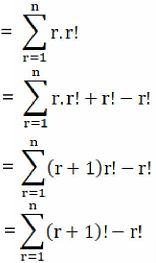= (2! – 1!) + (3! – 2!) + (4! – 3!) + ……… + (n! – (n – 1)!) + ((n+1)! – n!)

= 2! – 1! + 3! – 2! + 4! – 3! + ……… + n! – (n – 1)! + (n+1)! – n!

= (n + 1)! – 1!

= (n + 1)! – 1 [Since, P (n, n) = n!]

= P(n+1, n+1) – 1

= RHS

Hence Proved.

13. If P(15, r – 1) : P(16, r – 2) = 3 : 4, find r.

Solution:

Given:

P(15, r – 1) : P(16, r – 2) = 3 : 4

P(15, r – 1) / P(16, r – 2) = 3/4

By using the formula,

P (n, r) = n!/(n – r)!

P (15, r – 1) = 15! / (15 – r + 1)!

= 15! / (16 – r)!

P (16, r – 2) = 16!/(16 – r + 2)!

= 16!/(18 – r)!

So, from the question,

P(15, r – 1) / P(16, r – 2) = 3/4

Substituting the obtained values in above expression we get,

[15! / (16 – r)!] / [16!/(18 – r)!] = 3/4

[15! / (16 – r)!] × [(18 – r)! / 16!] = 3/4

[15! / (16 – r)!] × [(18 – r) (18 – r – 1) (18 – r – 2)!]/(16×15!) = 3/4

1/(16 – r)! × [(18 – r) (17 – r) (16 – r)!]/16 = 3/4

(18 – r) (17 – r) = 3/4 × 16

(18 – r) (17 – r) = 12

306 – 18r – 17r + r2 = 12

306 – 12 – 35r + r2 = 0

r2 – 35r + 294 = 0

r2 – 21r – 14r + 294 = 0

r(r – 21) – 14(r – 21) = 0

(r – 14) (r – 21) = 0

r = 14 or 21

For, P(n, r): r ≤ n

∴ r = 14 [for, P(15, r – 1)]

14. n+5Pn+1 = 11(n – 1)/2 n+3Pn, find n.

Solution:

Given:

n+5Pn+1 = 11(n – 1)/2 n+3Pn

P (n +5, n + 1) = 11(n – 1)/2 P(n + 3, n)

By using the formula,

P (n, r) = n!/(n – r)!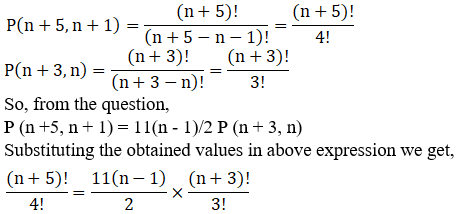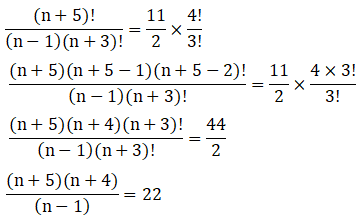(n + 5) (n + 4) = 22 (n – 1)

n2 + 4n + 5n + 20 = 22n – 22

n2 + 9n + 20 – 22n + 22 = 0

n2 – 13n + 42 = 0

n2 – 6n – 7n + 42 = 0

n(n – 6) – 7(n – 6) = 0

(n – 7) (n – 6) = 0

n = 7 or 6

∴ The value of n can either be 6 or 7.

15. In how many ways can five children stand in a queue?

Solution:

Number of arrangements of ‘n’ things taken all at a time = P (n, n)

So by using the formula,

By using the formula,

P (n, r) = n!/(n – r)!

The total number of ways in which five children can stand in a queue = the number of arrangements of 5 things taken all at a time = P (5, 5)

So,

P (5, 5) = 5!/(5 – 5)!

= 5!/0!

= 5! [Since, 0! = 1]

= 5 × 4 × 3 × 2 × 1

= 120

Hence, Number of ways in which five children can stand in a queue are 120.

16. From among the 36 teachers in a school, one principal and one vice-principal are to be appointed. In how many ways can this be done?

Solution:

Given:

The total number of teachers in a school = 36

We know, number of arrangements of n things taken r at a time = P(n, r)

By using the formula,

P (n, r) = n!/(n – r)!

∴ The total number of ways in which this can be done = the number of arrangements of 36 things taken 2 at a time = P(36, 2)

P (36, 2) = 36!/(36 – 2)!

= 36!/34!

= (36 × 35 × 34!)/34!

= 36 × 35

= 1260

Hence, Number of ways in which one principal and one vice-principal are to be appointed out of total 36 teachers in school are 1260.